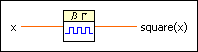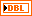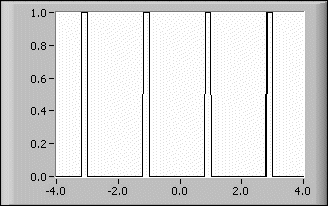# Square Function VI

## LabVIEW 2018 Help

Edition Date: March 2018
Part Number: 371361R-01
View Product InfoLabVIEW 2016 HelpLabVIEW 2017 HelpLabVIEW 2018 HelpLabVIEW 2019 HelpLabVIEW 2020 Help

Owning Palette: Gating Functions VIs

Requires: Full Development System

Generates the square function for any real number x.x is any real number.square(x) is the value of square(x) for the given value of x.

## Square Function Details

The following equation defines the square function.

square(x) = 1, if 2nx < 2n + 1 and 0 elsewhere,

where n is an integer.Note  You can define more complex functions by varying and combining the Step Function, Spike Function, and Square Function VIs, respectively.

The following illustration shows the graph for square(x) * square(x – 0.8) in the interval (–4.0, 4.0).